Next: Electrostatic Energy Up: Electrostatic Fields Previous: Coulomb's Law

# Electric Scalar Potential

It is easily demonstrated that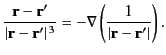(153)

Hence, Equation (152) yields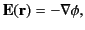(154)

where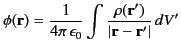(155)

is the scalar potential. (See Section 1.3.) It follows from Equation (154) that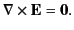(156)

In other words, an electric field generated by (stationary) charges is irrotational.

According to Equation (153), we can write Equation (152) in the form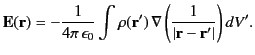(157)

Hence,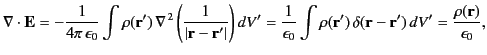(158)

where use has been made of Equations (23)-(25). We deduce that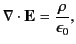(159)

which we recognize as the first Maxwell equation. (See Section 1.2.) The integral form of this equation, which follows from the divergence theorem,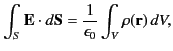(160)

is known as Gauss' law. Here,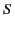is the bounding surface of volume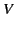.

Equations (154) and (159) can be combined to give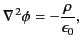(161)

which we recognize as Poisson's equation, with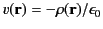. (See Section 2.3.) Hence, Equation (147) yields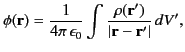(162)

which is equivalent to Equation (155). Incidentally, according to the analysis of Sections 2.2 and 2.3, the previous expression represents the unique solution to Equation (161), subject to the boundary condition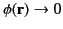as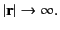(163)Next: Electrostatic Energy Up: Electrostatic Fields Previous: Coulomb's Law
Richard Fitzpatrick 2014-06-27